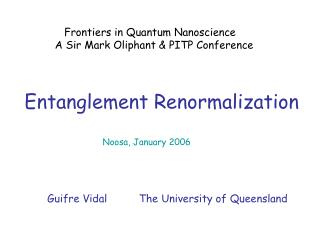DownloadDownload PresentationEntanglement Renormalization

Entanglement Renormalization

Download PresentationEntanglement Renormalization

- - - - - - - - - - - - - - - - - - - - - - - - - - - E N D - - - - - - - - - - - - - - - - - - - - - - - - - - -
Presentation Transcript

1. Frontiers in Quantum Nanoscience A Sir Mark Oliphant & PITP Conference Entanglement Renormalization Noosa, January 2006 Guifre Vidal The University of Queensland

2. Science and Technology of quantum many-body systems simulation algorithms entanglement Computational Physics Quantum Information Theory Introduction

3. Outline • Overview: new simulation algorithms for quantum systems • Time evolution in 1D quantum lattices (e.g. spin chains) • Entanglement renormalization

4. 2D 2005 1D Entanglement renormalization PEPS • Verstraete • Cirac 2004 2D TEBD 2003 time evolution ground state 1D 1D DMRG • White 1992 Recent results • Hastings • Osborne time (Other tools: mean field, density functional theory, quantum Monte Carlo, positive-P representation...)

5. N = 100 dim(H) = Computational problem • Simulating N quantum systems on a classical computer seems to be hard Hilbert Space dimension = 16 2 4 8 “small” system to test 2D Heisenberg model (High-T superconductivity) Hilbert Space dimension = 1,267,650,600,228,229,401,496,703,205,376

6. problems: solutions: (i) state • Use a tensor network: coefficients (for 1D systems MPS, DMRG) i1 ... in i1 ... in i1 in … (ii) evolution • Decompose it into small gates: (if , with ) coefficients i1 ... in i1 in … jn j1 … j1 ... jn

7. efficient description of and Trotter expansion matrix product state i1 ... in • efficient update of i1 ... in = operations j1 ... jn simulation of time evolution in 1D quantum lattices (spin chains, fermions, bosons,...)

8. tensor network (1D: matrix product state) i1 in … i1 in … coefficients coefficients coef efficiency entanglement A B Entanglement & efficient simulations • Schmidt decomposition

9. number of shared singlets A:B Entanglement in 1D systems • Toy model I (non-critical chain): correlation length • Toy model II (critical chain):

10. arbitrary state spins coefficients • non-critical 1D coefficients spins • critical 1D spins coefficients change of attitude summary: In DMRG, TEBD & PEPS, the amount of entanglement determines the efficiency of the simulation Entanglement renormalization disentangle the system

11. Entanglement renormalization no disentanglement partial disentanglement Examples: complete disentanglement

12. Entanglement renormalization Multi-scale entanglement renormalization ansatz (MERA)

13. C, fortran, highly optimized days in “big” machine a few hours in this laptop matlab Performance: system size (1D) time code memory greatest achievements in 13 years according to S. White DMRG ( ) Entanglement renormalization ( ) first tests at UQ Extension to 2D: work in progress

14. Understanding the structure of entanglement in • quantum many-body systems is the key to achieving • an efficient simulation in a wide range of problems. or simply... • There are new tools to efficiently simulate quantum • lattice systems in 1D, 2D, ... Conclusions